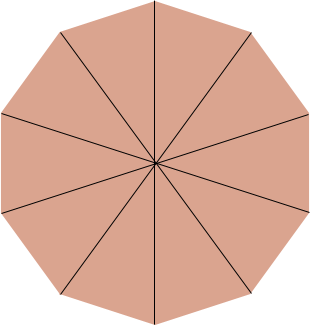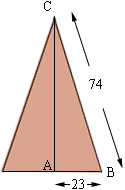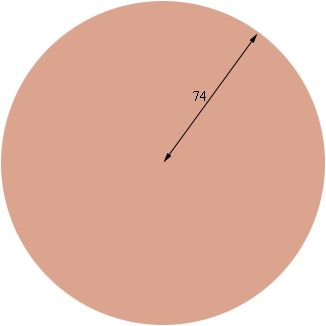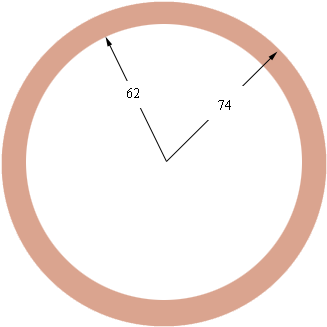Quandaries and Queries We are starting up a new business and for the business we will be required to order concrete for various shapes. Right now we have a job that requires a concrete slab that a decagon it is 140-3/4" from flat side to flat side, 74" from point to center and each flat side is 46" The pad should be 4" thick with 12"x12" continuous footing. I remember learning some of this stuff in college algebra however I have been out of school more years then I am willing to admit. I thank you in advance for your help and look forward to hearing from you soon. Tanya Hi Tanya, I will calculate the volume of the slab you describe and then show you a way to estimate the volume that is easier and probably "good enough". Below is a diagram of a decagon. When you draw the diagonals you can see that it can be divided into ten isosceles triangles.Each triangle has base 46 inches and side length of 74 inches. I drew one such triangle below.Pythagora's Theorem applied to the triangle ABC gives |AC| = Sqrt[742 - 232 ] = 70.33 inches. Thus the area of each of the ten triangles that make up the decagon is  1/2baseheight = 1/24670.33 = 1617.70 square inches. Thus the volume of the concrete slab is 101617.704 = 64708 cubic inches. One yard is 36 inches so the volume, in cubic yards is  64708/(363636) = 1.39 cubic yards. My suggestion for an estimation of this volume is to imagine that the concrete slab is a circle of radius 74 inches and thickness 4 inches.The volume of this slab isr2thickness =7424 = 68813.44 cubic inches which is  68813.44/(363636) = 1.47 cubic yards. The difference between 1.39 and 1.47 cubic yards is quite small and the second calculation is much easier, so I would suggest that for other slabs you use the circle approximation. By "with 12"x12" continuous footing" I assume you are describing a footing around the perimeter that is 12 inches wide and 12 inches deep. Again I am going to approximate by a circular slab.The area of the top of the footing is the area of the outside circle minus the area of the inside circle. That is742 -622 square inches and hence the volume is (742 -622 )12 = 61524.95 cubic inches or  61524.95/(363636) = 1.32 cubic yards. It is my experience that the smallest amount of concrete you can order is half a cubic yard so you will need one and a half cubic yards for the footing and then another one and a half yards for the pad. Harley Go to Math Central